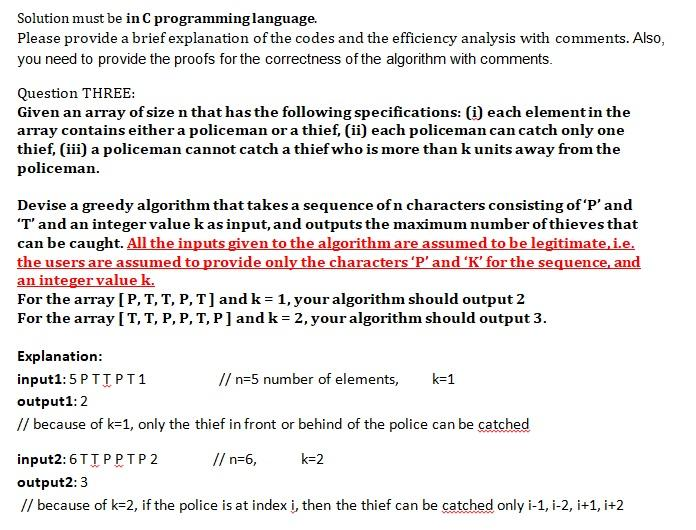# Question Solved1 AnswerFULL solution for this please. Solution must be in C programming language. Please provide a brief explanation of the codes and the efficiency analysis with comments. Also, you need to provide the proofs for the correctness of the algorithm with comments. Question THREE: Given an array of size n that has the following specifications: (i) each element in the array contains either a policeman or a thief, (ii) each policeman can catch only one thief, (iii) a policeman cannot catch a thief who is more than k units away from the policeman. Devise a greedy algorithm that takes a sequence of n characters consisting of'P'and 'T' and an integer value k as input, and outputs the maximum number of thieves that can be caught. All the inputs given to the algorithm are assumed to be legitimate, i.e. the users are assumed to provide only the characters 'P' and 'K' for the sequence, and an integer value k. For the array [P, T, T, P, T) and k = 1, your algorithm should output 2 For the array [T, T, P, P, T, P) and k = 2, your algorithm should output 3. k=1 Explanation: input1:5PTIPT1 // n=5 number of elements, output1:2 // because of k=1, only the thief in front or behind of the police can be catched input2:6TIPPTP 2 // n=6, k=2 output2:3 // because of k=2, if the police is at index i, then the thief can be catched only i-1, 1-2, i+1,i+2OJTPQT The Asker · Computer ScienceTranscribed Image Text: Solution must be in C programming language. Please provide a brief explanation of the codes and the efficiency analysis with comments. Also, you need to provide the proofs for the correctness of the algorithm with comments. Question THREE: Given an array of size n that has the following specifications: (i) each element in the array contains either a policeman or a thief, (ii) each policeman can catch only one thief, (iii) a policeman cannot catch a thief who is more than k units away from the policeman. Devise a greedy algorithm that takes a sequence of n characters consisting of'P'and 'T' and an integer value k as input, and outputs the maximum number of thieves that can be caught. All the inputs given to the algorithm are assumed to be legitimate, i.e. the users are assumed to provide only the characters 'P' and 'K' for the sequence, and an integer value k. For the array [P, T, T, P, T) and k = 1, your algorithm should output 2 For the array [T, T, P, P, T, P) and k = 2, your algorithm should output 3. k=1 Explanation: input1:5PTIPT1 // n=5 number of elements, output1:2 // because of k=1, only the thief in front or behind of the police can be catched input2:6TIPPTP 2 // n=6, k=2 output2:3 // because of k=2, if the police is at index i, then the thief can be catched only i-1, 1-2, i+1,i+2
More
Transcribed Image Text: Solution must be in C programming language. Please provide a brief explanation of the codes and the efficiency analysis with comments. Also, you need to provide the proofs for the correctness of the algorithm with comments. Question THREE: Given an array of size n that has the following specifications: (i) each element in the array contains either a policeman or a thief, (ii) each policeman can catch only one thief, (iii) a policeman cannot catch a thief who is more than k units away from the policeman. Devise a greedy algorithm that takes a sequence of n characters consisting of'P'and 'T' and an integer value k as input, and outputs the maximum number of thieves that can be caught. All the inputs given to the algorithm are assumed to be legitimate, i.e. the users are assumed to provide only the characters 'P' and 'K' for the sequence, and an integer value k. For the array [P, T, T, P, T) and k = 1, your algorithm should output 2 For the array [T, T, P, P, T, P) and k = 2, your algorithm should output 3. k=1 Explanation: input1:5PTIPT1 // n=5 number of elements, output1:2 // because of k=1, only the thief in front or behind of the police can be catched input2:6TIPPTP 2 // n=6, k=2 output2:3 // because of k=2, if the police is at index i, then the thief can be catched only i-1, 1-2, i+1,i+2